Friday, October 22, 2021
Home > IMO > Pre RMO Previous Year 2016 Question Paper With Solutions

# Pre RMO Previous Year 2016 Question Paper With Solutions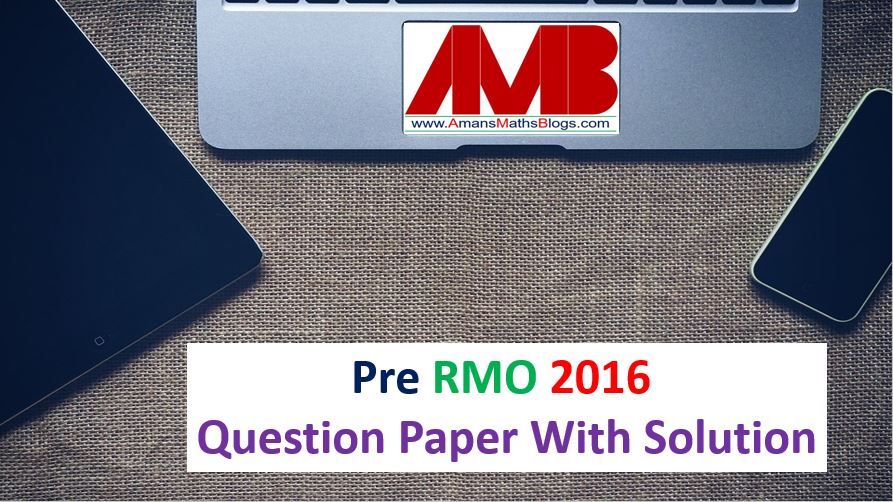# Pre RMO Previous Year 2016 Question Paper With Solutions

In this post, you will get Pre RMO Previous Year 2016 Question Paper With Solutions.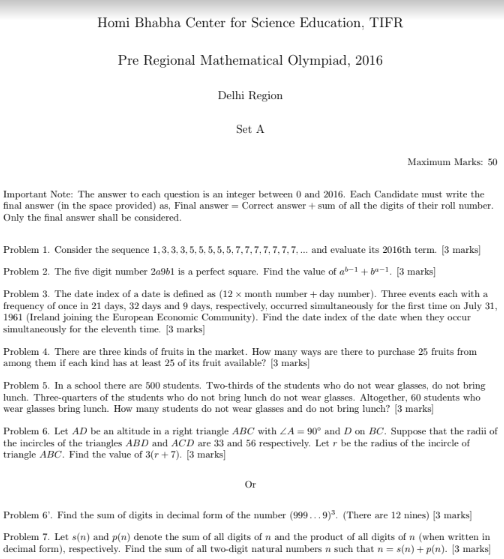Pre RMO Previous Year 2016 Question Paper Question No 1:

Consider the sequence 1, 3, 3, 3, 5, 5, 5, 5, 5, 7, 7, 7, 7, 7, 7, 7, … and evaluate its 2016th term.

Pre RMO Previous Year 2016 Question Paper Solution of Question No 1: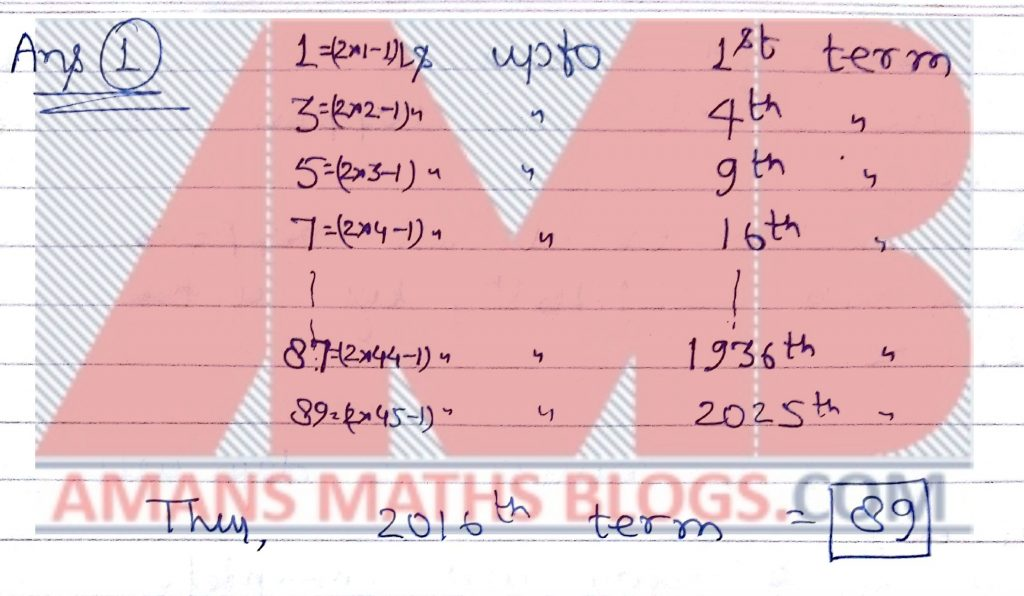Pre RMO Previous Year 2016 Question Paper Question No 2:

The five digit number 2a9b1 is a perfect square. Find the value of ab−1 + ba−1.

Pre RMO Previous Year 2016 Question Paper Solution of Question No 2: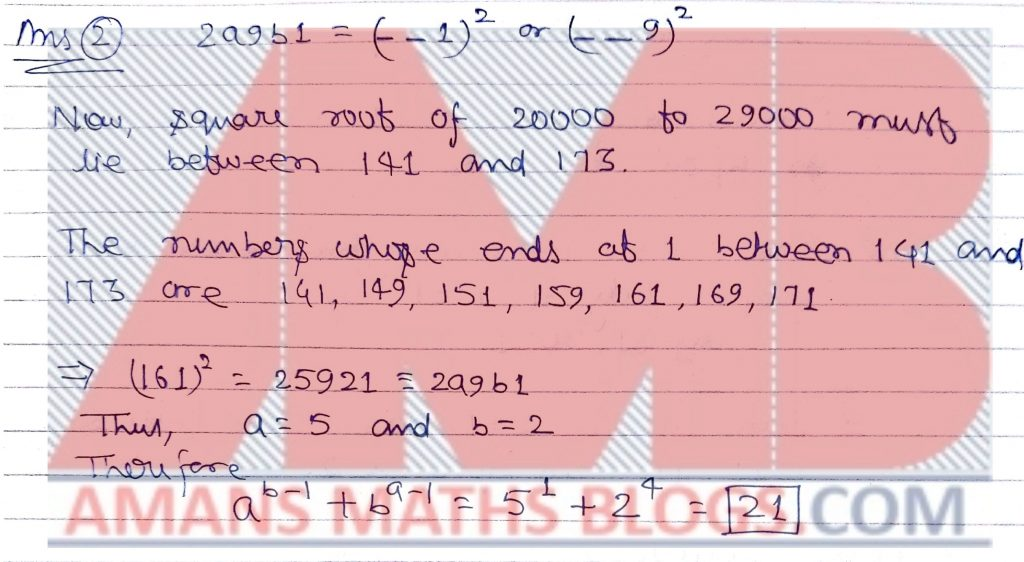Pre RMO Previous Year 2016 Question Paper Question No 3:

The date index of a date is defined as (12 × month number + day number). Three events each with a frequency of once in 21 days, 32 days and 9 days, respectively, occurred simultaneously for the first time on July 31, 1961 (Ireland joining the European Economic Community). Find the date index of the date when they occur simultaneously for the eleventh time.

Pre RMO Previous Year 2016 Question Paper Solution of Question No 3: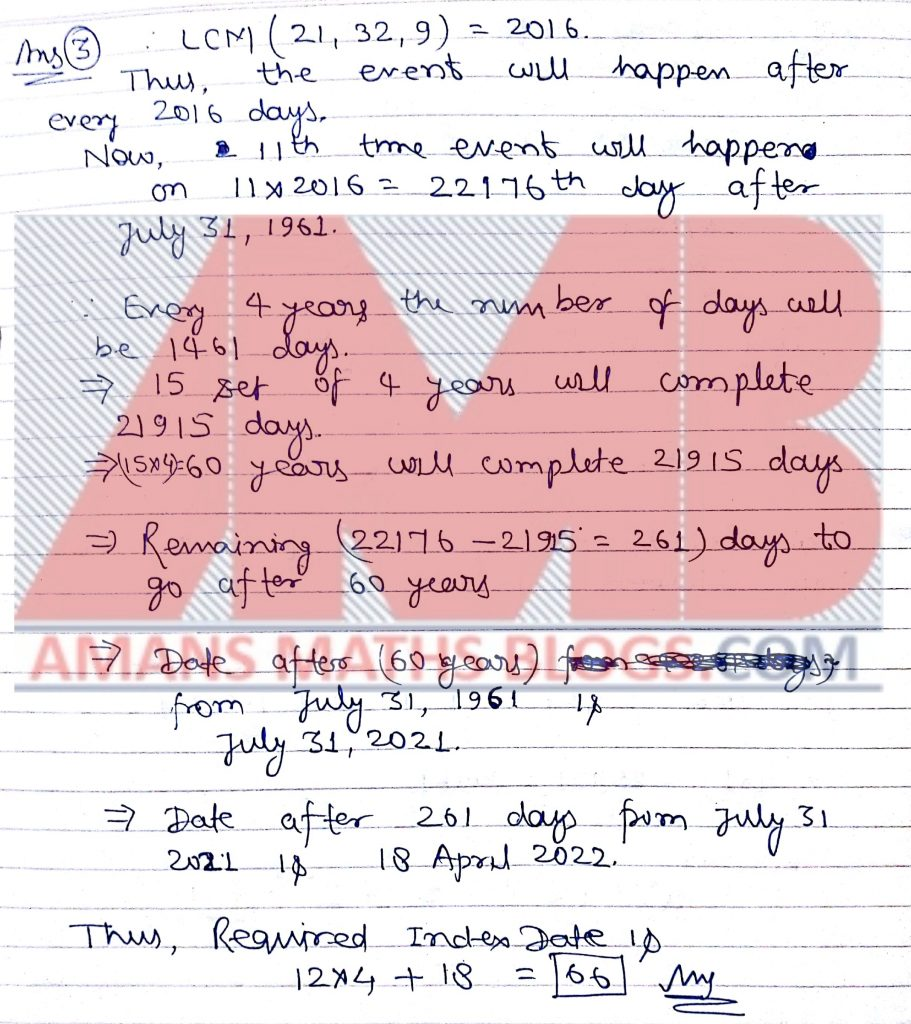Pre RMO Previous Year 2016 Question Paper Question No 4:

There are three kinds of fruits in the market. How many ways are there to purchase 25 fruits from among them if each kind has at least 25 of its fruit available?

Pre RMO Previous Year 2016 Question Paper Solution of Question No 4: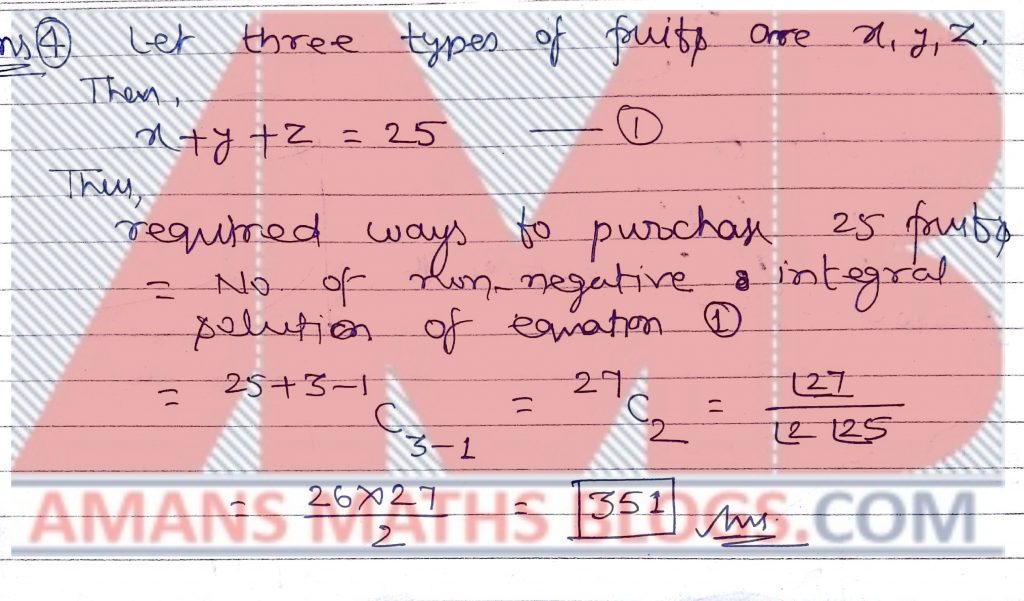Pre RMO Previous Year 2016 Question Paper Question No 5:

In a school there are 500 students. Two-thirds of the students who do not wear glasses, do not bring lunch. Three-quarters of the students who do not bring lunch do not wear glasses. Altogether, 60 students who wear glasses bring lunch. How many students do not wear glasses and do not bring lunch?

Pre RMO Previous Year 2016 Question Paper Solution of Question No 5: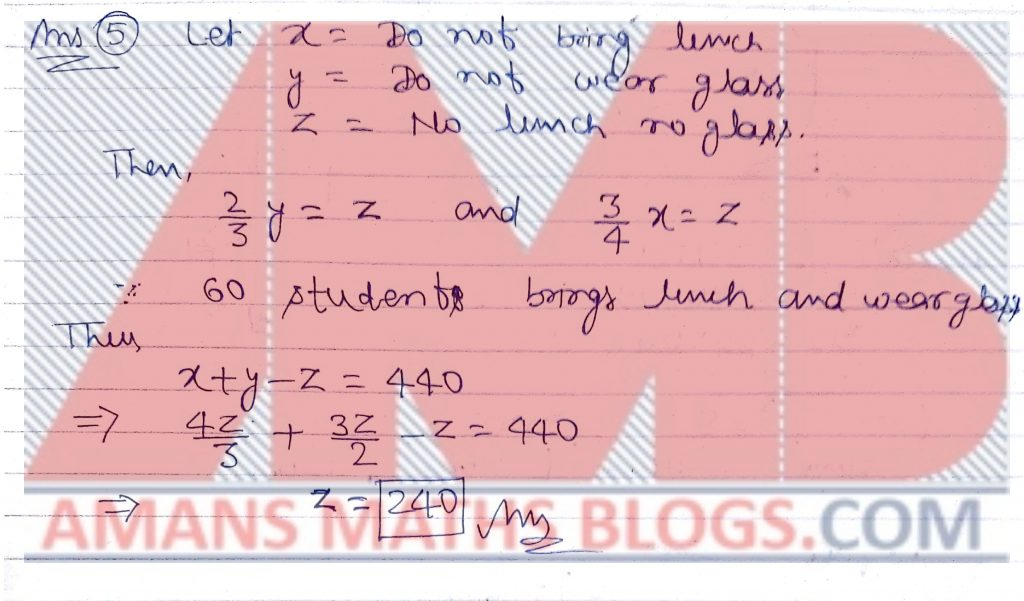Pre RMO Previous Year 2016 Question Paper Question No 6:

Find the sum of digits in decimal form of the number (999 . . . 9)3 . (There are 12 nines).

Pre RMO Previous Year 2016 Question Paper Solution of Question No 6: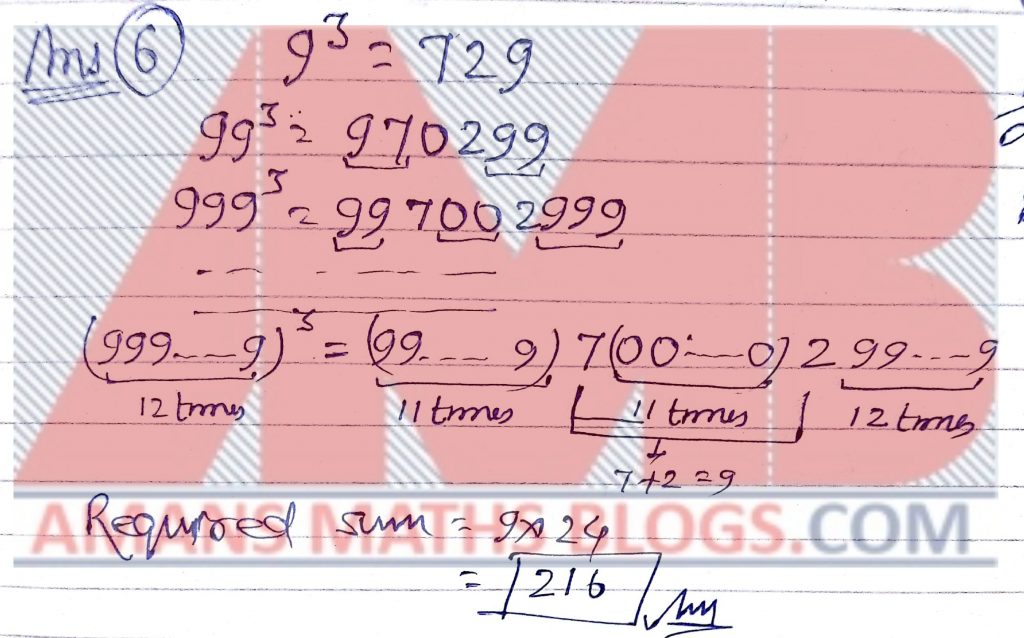Pre RMO Previous Year 2016 Question Paper Question No 7:

Let s(n) and p(n) denote the sum of all digits of n and the product of all digits of n (when written in decimal form), respectively. Find the sum of all two-digit natural numbers n such that n = s(n) + p(n).

Pre RMO Previous Year 2016 Question Paper Solution of Question No 7: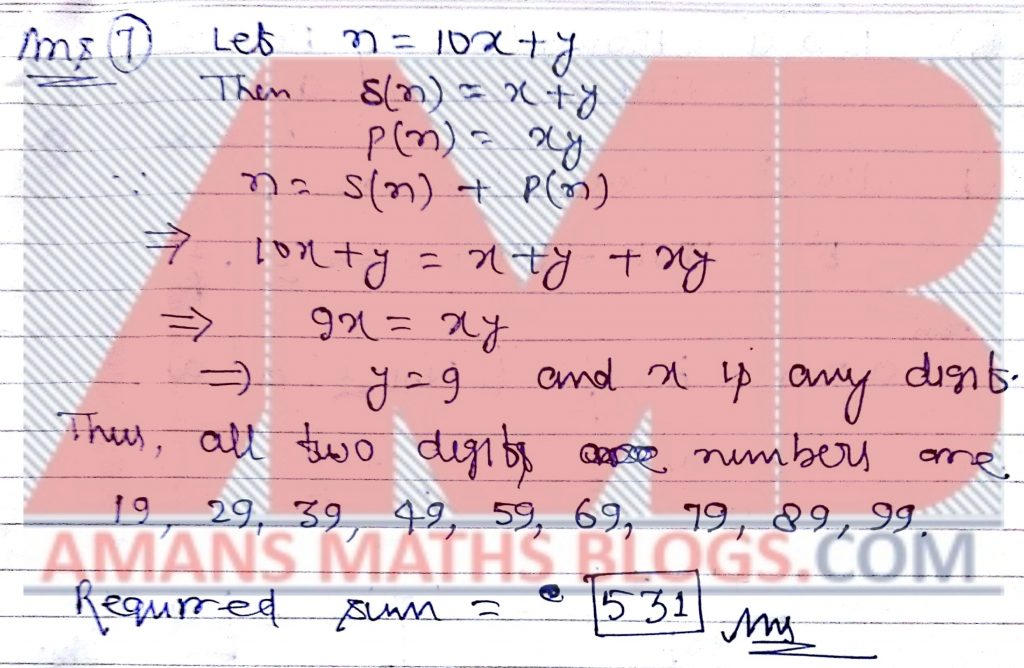Pre RMO Previous Year 2016 Question Paper Question No 8:

Suppose that a and b are real numbers such that ab ≠ 1 and the equations 120a2 − 120a + 1 = 0 and b2 − 120b + 120 = 0 hold. Find the value of.

Pre RMO Previous Year 2016 Question Paper Solution of Question No 8: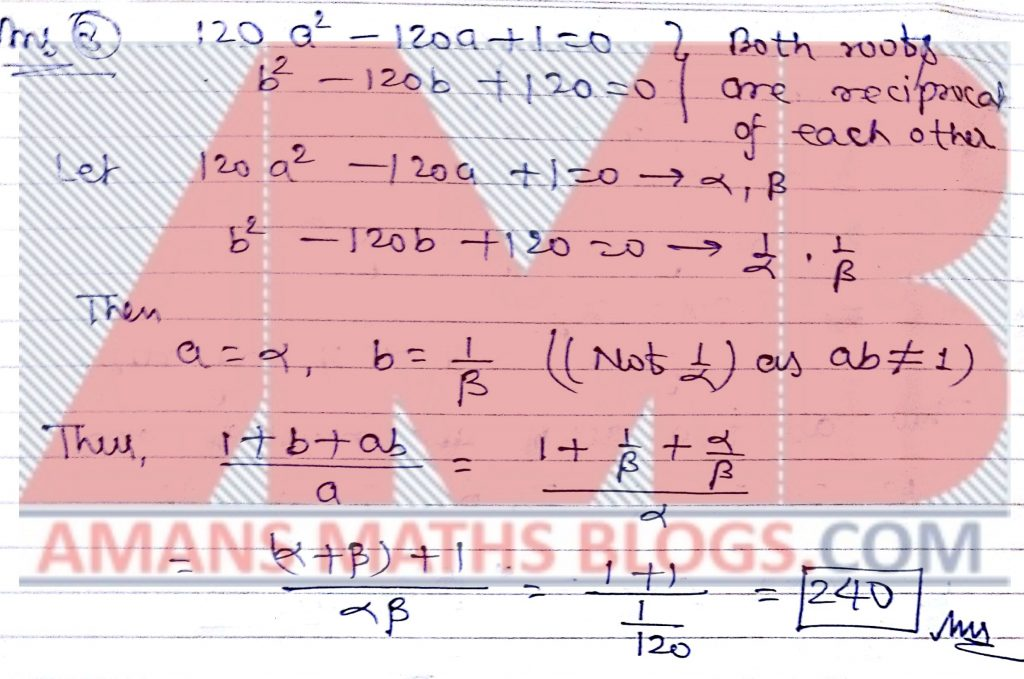Pre RMO Previous Year 2016 Question Paper Question No 9:

Between 5pm and 6pm, I looked at my watch. Mistaking the hour hand for the minute hand and the minute hand for the hour hand, I mistook the time to be 57 minutes earlier than the actual time. Find the number of minutes past 5 when I looked at my watch.

Pre RMO Previous Year 2016 Question Paper Solution of Question No 9: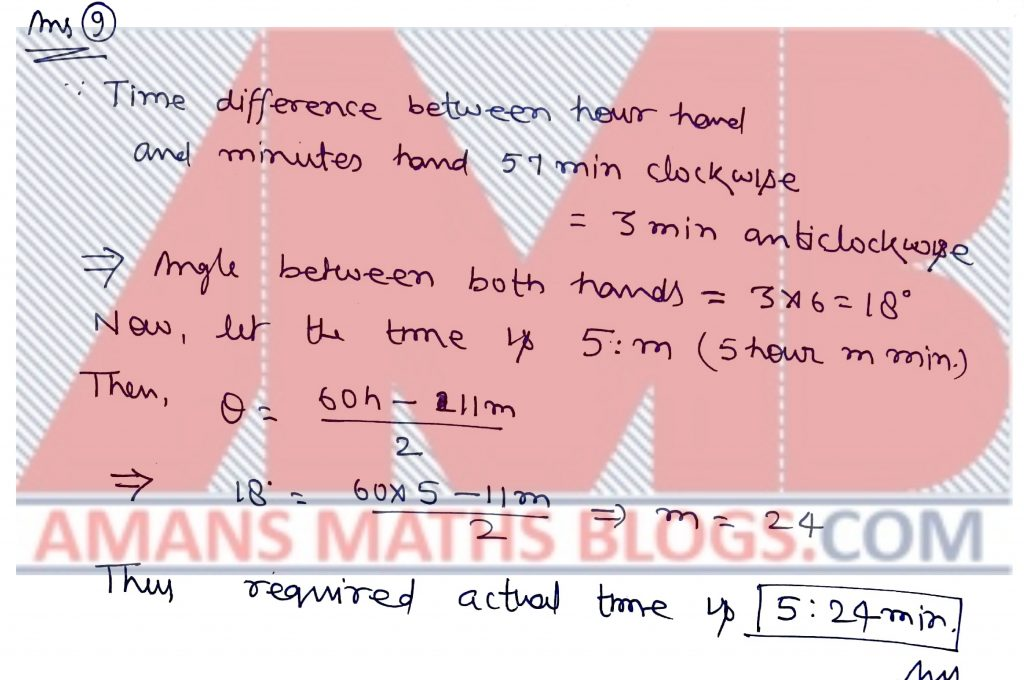Pre RMO Previous Year 2016 Question Paper Question No 10:

In triangle ABC right angled at vertex B, a point O is chosen on the side BC such that the circle γ centered at O of radius OB touches the side AC. Let AB = 63 and BC = 16, and the radius of γ be of the form m n where m, n are relatively prime positive integers. Find the value of m + n.

Pre RMO Previous Year 2016 Question Paper Solution of Question No 10: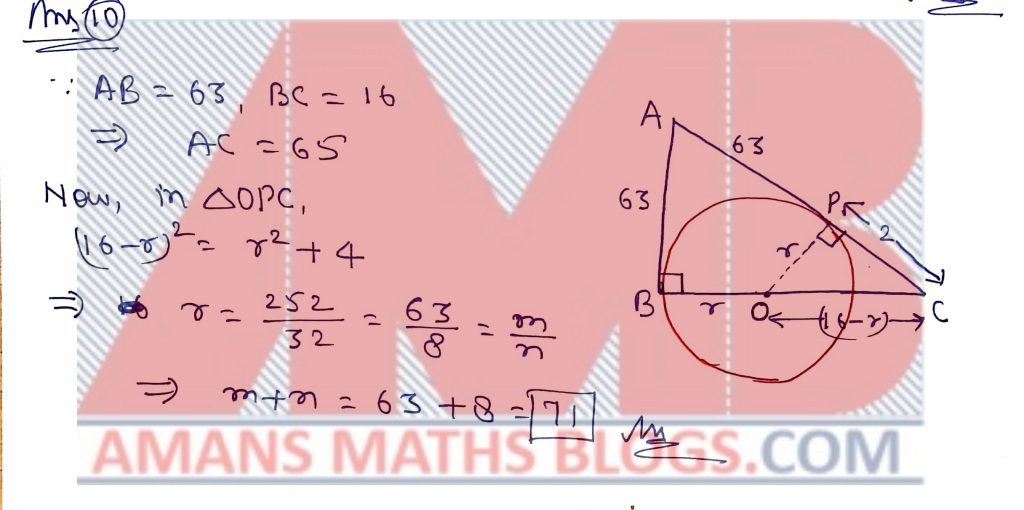Pre RMO Previous Year 2016 Question Paper Question No 11:

Consider the 50 term sums: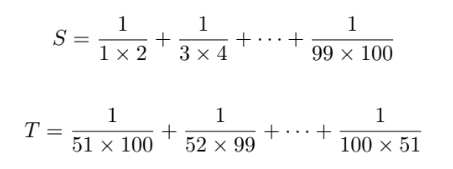The ratiois written in the lowest formwhere m, n are relatively prime natural numbers. Find the value of

m + n.

Pre RMO Previous Year 2016 Question Paper Solution of Question No 11: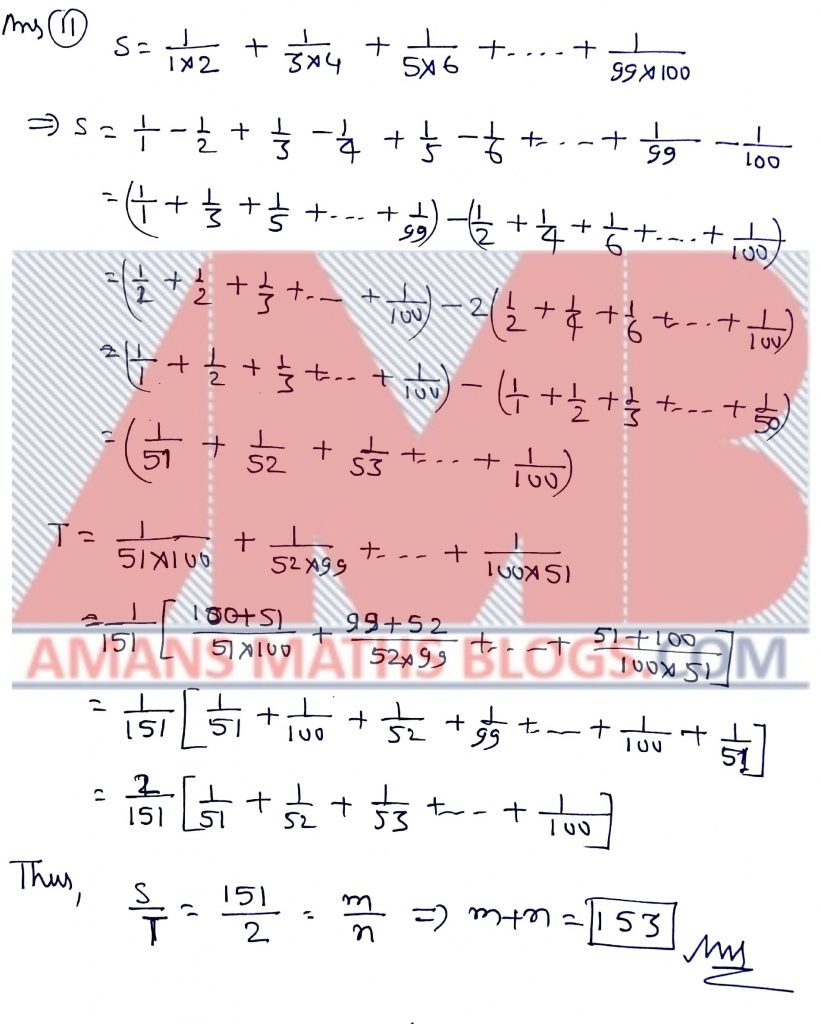Pre RMO Previous Year 2016 Question Paper Question No 12:

Find the value of the expression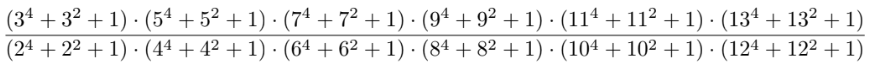when written in lowest form.

Pre RMO Previous Year 2016 Question Paper Solution of Question No 12: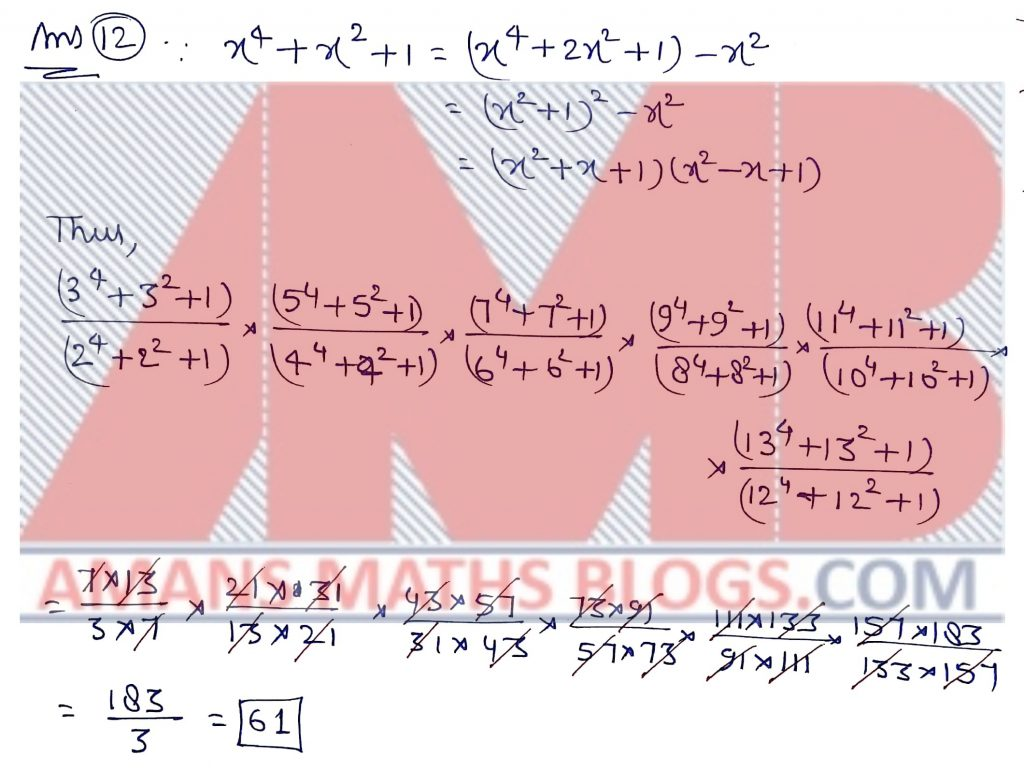Pre RMO Previous Year 2016 Question Paper Question No 13:

The hexagon OLY MP I has a reflex angle at O and convex at every other vertex. Suppose that LP = 3√ 2 units and the condition ∠O = 10∠L = 2∠Y = 5∠M = 2∠P = 10∠I hold. Find the area (in sq units) of the hexagon.

Pre RMO Previous Year 2016 Question Paper Solution of Question No 13:

Pre RMO Previous Year 2016 Question Paper Question No 14:

A natural number a has four digits and a 2 ends with the same four digits as that of a. Find the value of (10080 − a).

Pre RMO Previous Year 2016 Question Paper Solution of Question No 14: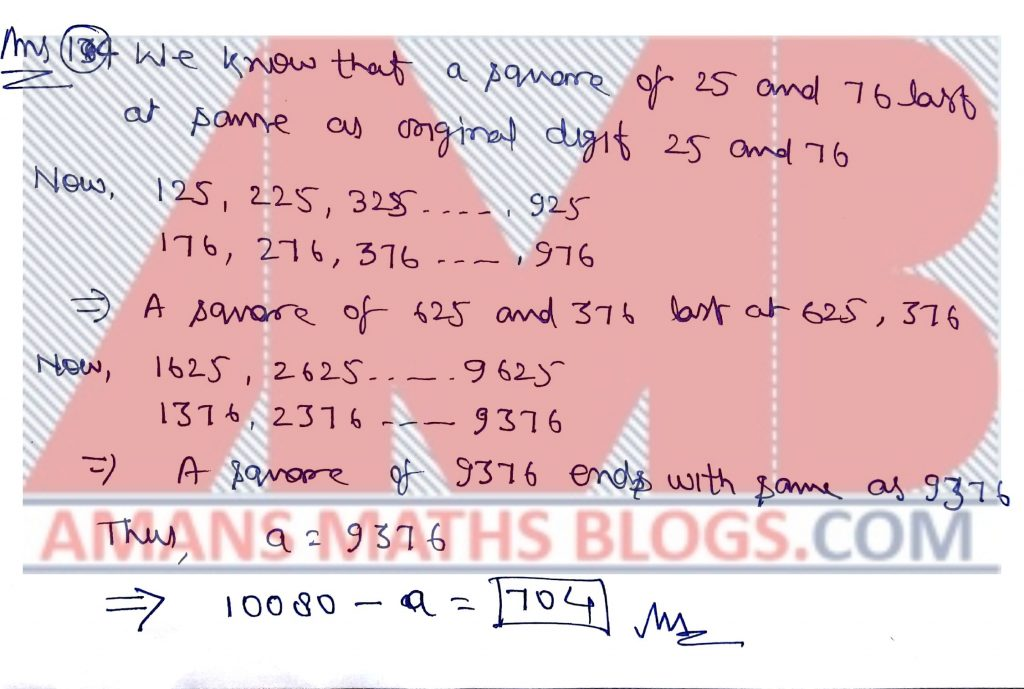Pre RMO Previous Year 2016 Question Paper Question No 15:

Points G and O denote the centroid and the circumcenter of the triangle ABC. Suppose that ∠AGO = 90◦ and AB = 17, AC = 19. Find the value of BC2.

Pre RMO Previous Year 2016 Question Paper Solution of Question No 15: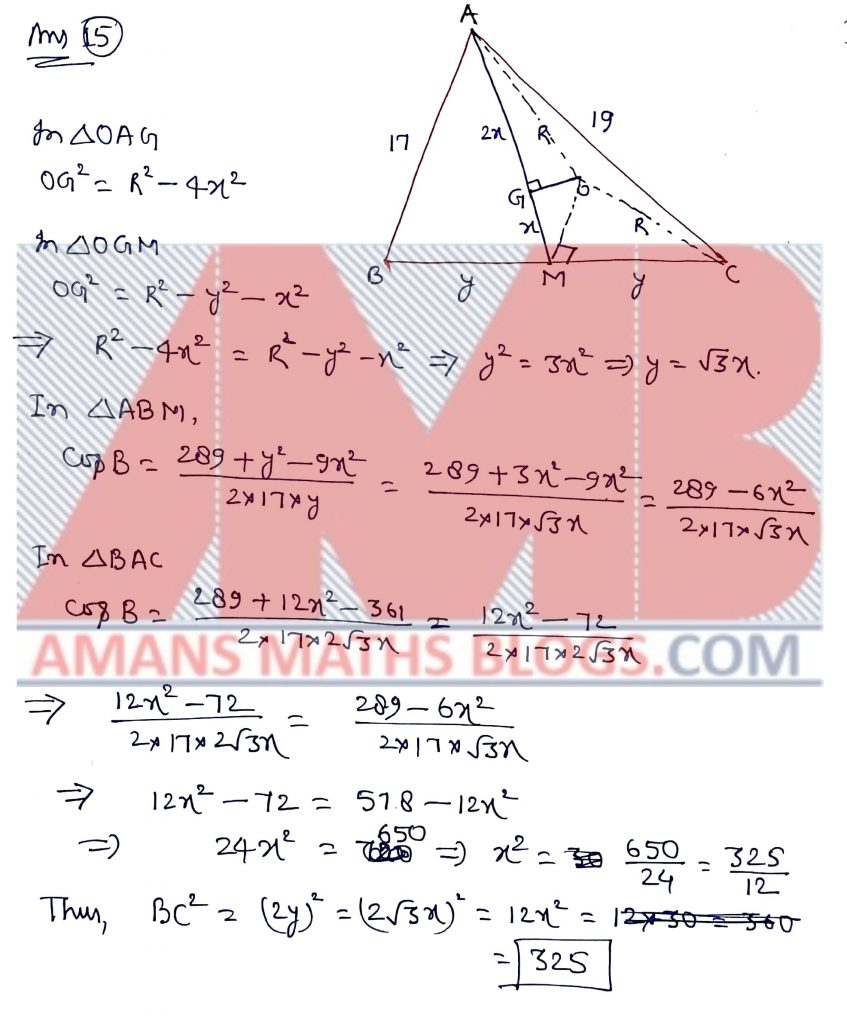Pre RMO Previous Year 2016 Question Paper With SolutionGet Combined Pre RMO 2012 to 2018 Questions Paper with Solution (Click on Image)
AMBiPi
error: Content is protected !!